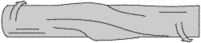# buckling

(redirected from bucklings)
Also found in: Dictionary, Medical.

## buckling

[′bək·liŋ]
(engineering)
Wrinkling or warping of fibers in a composite material.
(mechanics)
Bending of a sheet, plate, or column supporting a compressive load.
(nucleonics)
The size-shape factor that appears in the general nuclear reactor equation and is a measure of the curvature of the neutron density distribution in the reactor.
McGraw-Hill Dictionary of Scientific & Technical Terms, 6E, Copyright © 2003 by The McGraw-Hill Companies, Inc.
The following article is from The Great Soviet Encyclopedia (1979). It might be outdated or ideologically biased.

## Buckling

in strength of materials, the bending of an originally straight column under the effect of centrally applied axial compressive forces that exceed the column’s bearing power. For a column of uniform cross section exhibiting elastic behavior, the various forms of buckling correspond to critical values of the compressive forces Nc = μ2n2EI/(μl)2, where E is the modulus of elasticity of the material of the column, I is the minimum value of the axial moment of inertia of the column’s cross section, l is the length of the column, μ is the coefficient of reduced length dependent on the conditions of end support of the column, and n is an integer. The minimum value for the critical force is usually of practical interest in that for a column with pinned ends (μ = 1), this force causes bending of the column according to a half-cycle sine curve (n = 1). The magnitude of the force is calculated by the Euler formula Nc = π2EI/l2. The stress σc = Nc/F(F is the cross-sectional area of the column) corresponds to the critical force and is called the critical stress. If the value of the critical stress exceeds the proportional limit of the column material, then buckling occurs in the zone of plastic deformations. In this case, the minimum critical force is determined by the formula Nc = π2n2TI/(μl)2, where T is the modulus, which characterizes the dependence between the deformations and the stresses beyond the limits of the elastic deformations.

In structural design, buckling is taken into account in calculating column loads.

L. V. KASAB’IAN

## buckling

The collapse of a slender vertical element which has been subjected to compression, leading to a sudden sideways deflection.
McGraw-Hill Dictionary of Architecture and Construction. Copyright © 2003 by McGraw-Hill Companies, Inc.

## bucklingBuckling failure of hollow shaft of ductile material.
A state of unstable equilibrium of a thinwalled body when compressive loads are applied on its walls. The resultant deformation may be elastic (i.e., the body regains its shape once compressive loads are removed) or permanent. In some cases, it may even lead to collapse of the structure. The most visible forms of buckling are wrinkles or kinks on the surface of a sheet metal aircraft structure.